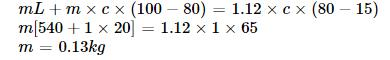Courses

# First Law Of Thermodynamic MCQ Level - 1 (Part - 1)

## 10 Questions MCQ Test Topic wise Tests for IIT JAM Physics | First Law Of Thermodynamic MCQ Level - 1 (Part - 1)

Description
This mock test of First Law Of Thermodynamic MCQ Level - 1 (Part - 1) for IIT JAM helps you for every IIT JAM entrance exam. This contains 10 Multiple Choice Questions for IIT JAM First Law Of Thermodynamic MCQ Level - 1 (Part - 1) (mcq) to study with solutions a complete question bank. The solved questions answers in this First Law Of Thermodynamic MCQ Level - 1 (Part - 1) quiz give you a good mix of easy questions and tough questions. IIT JAM students definitely take this First Law Of Thermodynamic MCQ Level - 1 (Part - 1) exercise for a better result in the exam. You can find other First Law Of Thermodynamic MCQ Level - 1 (Part - 1) extra questions, long questions & short questions for IIT JAM on EduRev as well by searching above.
QUESTION: 1

### A vessel contains 1 mole of O2 gas (molar mass 32) at a temperature T. The pressure of the gas is p. An identical vessels containing one mole of the gas (molar mass 4) at a temperature 2T has a pressure of

Solution:QUESTION: 2

### A gas mixture consists of 2 moles of oxygen and 4 moles of argon at temperature T. Neglecting all vibrational modes, the total internal energy of the system is

Solution:

Internal energy of n moles of an ideal gas at temperature T is given by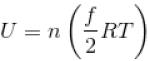where, f = degrees of freedom
= 5 for O3 and 3 for Ar  [∴ O2 is diatomic, Ar is monoatomic]
Hence,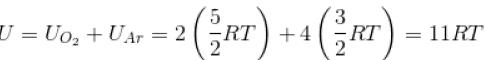The correct answer is: 11 RT

QUESTION: 3

### An ideal monoatomic gas is taken round that cycle ABCDA as shown in the p-V diagram (see figure). The work done during the cycle is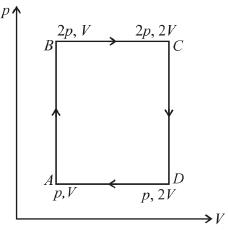Solution:

Work done in a cyclic process = area under the graph in p-V diagram
Area = AB × BC = (2p – p) × (2V – V) = pV
∴ So, work done = pV

QUESTION: 4

In a given process of an ideal gas, dW = 0 and dQ < 0. The for the gas
Select one:

Solution:

From first law of thermodynamics,
dQ = dU + dW
dQ = dU if dW = 0
Since, dQ < 0
Therefore,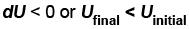or temperature will decrease
The correct answer is: the temperature will decrease

QUESTION: 5

70 cal of heat are required to raise the temperature of 2 moles of an ideal diatomic gas at constant pressure from 30ºC to 35°C. The amount of heat required (in calorie) to raise the temperature of the same gas through the same range (30°C to 35°C) at constant volume is

Solution:

We know that heat required to raise the temperature at constant pressure is Cp.
So,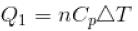Similarly, at constant volume CV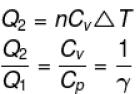or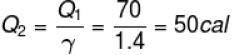QUESTION: 6

An ideal gas is initially at temperature T and volume V. Its volume is increased by ΔV  due to an increase in temperature ΔT  pressure remaining constant. The quantity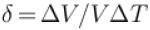varies with temperature as
Select one:

Solution:

For an ideal gas : pV = nRT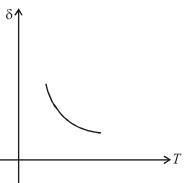For p = constant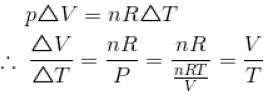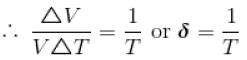Therefore,δ is inversely proportional to temperature T i.e., when T increases, δ decreases and vice-versa.
Hence,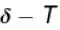graph will be rectangular hyperbola as shown in the above figure.
The correct answer is: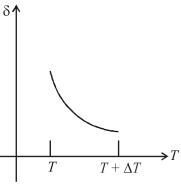QUESTION: 7

When an ideal diatomic gas is heated at constant pressure, the fraction of the heat energy supplied  which increases the internal energy of the gas is
Select one:

Solution: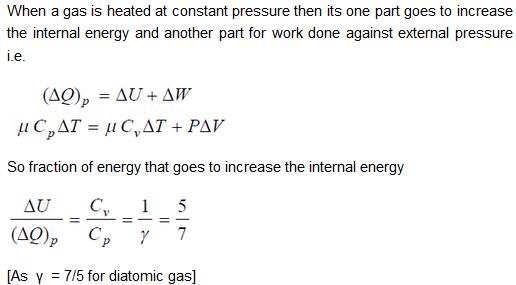QUESTION: 8

Starting with the same initial conditions, an ideal gas expands from volume V1 to V2 in three different ways, the work done by the gas is W1 if the process is purely isothermal, W2 if purely isobaric and W3 if purely adiabatic, then

Solution:

The corresponding p-V graph (also called indicator diagram) in three different process will be as shown :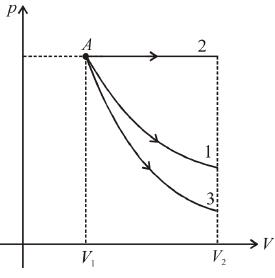Area under the graph gives the work done by the gas.
(Area)2 > (Area)1 > (Area)3
∴ W2 > W1 > W3
W2 > W1 > W3

QUESTION: 9

An ideal gas expands isothermally from a volume V1 to V2 and then compressed to original volumes V1 adiabatically. Initial pressure is p1 and final pressure is p3. The total work done is W. Then,
Select one:

Solution:

Slope of adiabatic process at a given state (p, V, T) is more than the slope of isothermal process. The corresponding p-V graph for the two process is as shown in figure.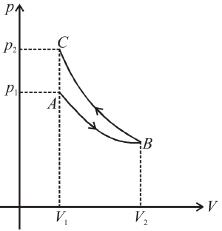In the graph, AB is isothermal and BC is adiabatic.
WAB is positive (as volume is increasing)
and WBC is negative (as volume is decreasing)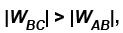as area under p–V graph gives the work done.
Hence,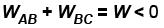From the graph itself, it is clear that p3 > p1
The correct answer is: p> p1 , W < 0

QUESTION: 10

Steam at 100°C is passed into 1.1 kg of water contained in a calorimeter of water equivalent 0.02 kg at 15°C till the temperature of the calorimeter and its contents rises to 80°C. The mass of the steam condensed (in kg) is
Select one:

Solution:

Heat lost by steam =Heat gained by
(water +calorimeter)